# 18 Results

View
Selected filters:
• WI.Math.Content.HSF-BF.B.3Conditional Remix & Share Permitted
CC BY-NC-SA
Rating

In this module, students synthesize and generalize what they have learned about a variety of function families.  They extend the domain of exponential functions to the entire real line (N-RN.A.1) and then extend their work with these functions to include solving exponential equations with logarithms (F-LE.A.4).  They explore (with appropriate tools) the effects of transformations on graphs of exponential and logarithmic functions.  They notice that the transformations on a graph of a logarithmic function relate to the logarithmic properties (F-BF.B.3).  Students identify appropriate types of functions to model a situation.  They adjust parameters to improve the model, and they compare models by analyzing appropriateness of fit and making judgments about the domain over which a model is a good fit.  The description of modeling as, the process of choosing and using mathematics and statistics to analyze empirical situations, to understand them better, and to make decisions, is at the heart of this module.  In particular, through repeated opportunities in working through the modeling cycle (see page 61 of the CCLS), students acquire the insight that the same mathematical or statistical structure can sometimes model seemingly different situations.

Subject:
Algebra
Material Type:
Module
Provider:
New York State Education Department
Provider Set:
EngageNY
Date Added:
09/16/2014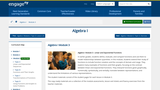Conditional Remix & Share Permitted
CC BY-NC-SA
Rating

In earlier grades, students define, evaluate, and compare functions and use them to model relationships between quantities. In this module, students extend their study of functions to include function notation and the concepts of domain and range. They explore many examples of functions and their graphs, focusing on the contrast between linear and exponential functions. They interpret functions given graphically, numerically, symbolically, and verbally; translate between representations; and understand the limitations of various representations.

Subject:
Algebra
Material Type:
Module
Provider:
New York State Education Department
Provider Set:
EngageNY
Date Added:
09/17/2013Conditional Remix & Share Permitted
CC BY-NC-SA
Rating

In earlier modules, students analyze the process of solving equations and developing fluency in writing, interpreting, and translating between various forms of linear equations (Module 1) and linear and exponential functions (Module 3). These experiences combined with modeling with data (Module 2), set the stage for Module 4. Here students continue to interpret expressions, create equations, rewrite equations and functions in different but equivalent forms, and graph and interpret functions, but this time using polynomial functions, and more specifically quadratic functions, as well as square root and cube root functions.

Subject:
Algebra
Material Type:
Module
Provider:
New York State Education Department
Provider Set:
EngageNY
Date Added:
09/17/2013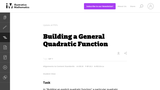Conditional Remix & Share Permitted
CC BY-NC-SA
Rating

This task is for instructional purposes only and builds on ``Building an explicit quadratic function.''

Subject:
Mathematics
Algebra
Functions
Material Type:
Activity/Lab
Provider:
Illustrative Mathematics
Provider Set:
Illustrative Mathematics
Author:
Illustrative Mathematics
Date Added:
08/20/2012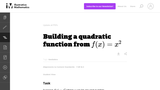Conditional Remix & Share Permitted
CC BY-NC-SA
Rating

This is the first of a series of task aiming at understanding the quadratic formula in a geometric way in terms of the graph of a quadratic function.

Subject:
Mathematics
Functions
Material Type:
Activity/Lab
Provider:
Illustrative Mathematics
Provider Set:
Illustrative Mathematics
Author:
Illustrative Mathematics
Date Added:
08/15/2012Conditional Remix & Share Permitted
CC BY-NC-SA
Rating

This task is intended for instruction and to motivate "Building a general quadratic function.''

Subject:
Mathematics
Functions
Material Type:
Activity/Lab
Provider:
Illustrative Mathematics
Provider Set:
Illustrative Mathematics
Author:
Illustrative Mathematics
Date Added:
08/26/2013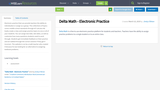Only Sharing Permitted
CC BY-NC-ND
Rating

Electronic practice that can provide teachers the ability to individualize or assign as a group. This collections of topics covers middle school standards through AP Calculus AB. Easily create a class and assign practice topics to one or all of your students. You can assign due date, late dates, as well as customize how many questions students need to work through. Students get immediate feedback on their practice and are walked through the correct solution if they get it incorrect. This website is run by a math teacher who created it because he was looking for an alternative to assigning textbook problems.

Subject:
Mathematics
Material Type:
Formative Assessment
Homework/Assignment
Interactive
Author:
Emily O'Brien
Date Added:
08/02/2019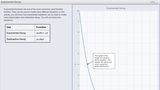Restricted Use
Copyright Restricted
Rating

In this activity students learn to use two different equations for modeling real-life situations that involve exponential decay. This is the third of three activities about Exponential Functions. The associated lesson plan and student assessment questions can be found at: https://concord.org/projects/smartgraphs#curriculum and clicking on Ã¢â‚¬Å“AlgebraÃ¢â‚¬Â and then Ã¢â‚¬Å“Exponential Functions.Ã¢â‚¬Â

Subject:
Mathematics
Material Type:
Activity/Lab
Diagram/Illustration
Formative Assessment
Interactive
Learning Task
Lesson Plan
Self Assessment
Simulation
Provider:
The Concord Consortium
Date Added:
12/12/2016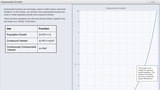Restricted Use
Copyright Restricted
Rating

Learn how equations for several exponential functions are used to model population growth and compound interest. This is the second of three activities about exponential functions. The associated lesson plan and student assessment questions can be found at: https://concord.org/projects/smartgraphs#curriculum and clicking on Ã¢â‚¬Å“AlgebraÃ¢â‚¬Â and then Ã¢â‚¬Å“Exponential Functions.Ã¢â‚¬Â

Subject:
Mathematics
Material Type:
Activity/Lab
Diagram/Illustration
Formative Assessment
Interactive
Learning Task
Lesson Plan
Self Assessment
Simulation
Provider:
The Concord Consortium
Date Added:
12/12/2016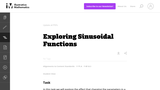Conditional Remix & Share Permitted
CC BY-NC-SA
Rating

This is a task from the Illustrative Mathematics website that is one part of a complete illustration of the standard to which it is aligned. Each task has at least one solution and some commentary that addresses important asects of the task and its potential use. Here are the first few lines of the commentary for this task: In this task we will explore the effect that changing the parameters in a sinusoidal function has on the graph of the function. A general sinusoidal fu...

Subject:
Mathematics
Material Type:
Activity/Lab
Provider:
Illustrative Mathematics
Provider Set:
Illustrative Mathematics
Author:
Illustrative Mathematics
Date Added:
01/06/2014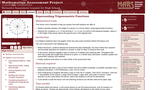Only Sharing Permitted
CC BY-NC-ND
Rating

This lesson unit is intended to help teachers assess how well students are able to: model a periodic situation, the height of a person on a Ferris wheel, using trigonometric functions; and interpret the constants a, b, c in the formula h = a + b cos ct in terms of the physical situation, where h is the height of the person above the ground and t is the elapsed time.

Subject:
Functions
Material Type:
Assessment
Lesson Plan
Provider:
Shell Center for Mathematical Education
Date Added:
11/01/2017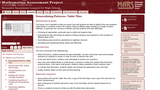Only Sharing Permitted
CC BY-NC-ND
Rating

This lesson unit is intended to help teachers assess how well students are able to identify linear and quadratic relationships in a realistic context: the number of tiles of different types that are needed for a range of square tabletops. In particular, this unit aims to identify and help students who have difficulties with: choosing an appropriate, systematic way to collect and organize data; examining the data and looking for patterns; finding invariance and covariance in the numbers of different types of tile; generalizing using numerical, geometrical or algebraic structure; and describing and explaining findings clearly and effectively.

Subject:
Algebra
Geometry
Measurement and Data
Material Type:
Assessment
Lesson Plan
Provider:
Shell Center for Mathematical Education
Date Added:
11/01/2017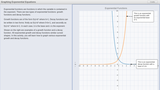Restricted Use
Copyright Restricted
Rating

Students graph exponential growth and decay functions by connecting ordered pairs. This is the first of three activities about Exponential Functions. The associated lesson plan and student assessment questions can be found at: https://concord.org/projects/smartgraphs#curriculum and clicking on Ã¢â‚¬Å“AlgebraÃ¢â‚¬Â and then Ã¢â‚¬Å“Exponential Functions.Ã¢â‚¬Â

Subject:
Mathematics
Material Type:
Activity/Lab
Diagram/Illustration
Formative Assessment
Interactive
Learning Task
Lesson Plan
Self Assessment
Simulation
Provider:
The Concord Consortium
Date Added:
12/12/2016Conditional Remix & Share Permitted
CC BY-NC-SA
Rating

In this task students use algebraic methods to determine whether each of the functions is odd, even, or neither.

Subject:
Mathematics
Functions
Material Type:
Activity/Lab
Provider:
Illustrative Mathematics
Provider Set:
Illustrative Mathematics
Author:
Illustrative Mathematics
Date Added:
05/01/2012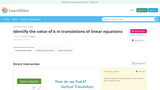Rating

This activity clearly shows students how to find the value of k in a traanslation of a linear function.  It shows the student the difference between a vertical and a horizontal translation.

Subject:
Mathematics
Material Type:
Lesson Plan
Simulation
Provider:
Learnzillion
Date Added:
03/20/2018Conditional Remix & Share Permitted
CC BY-NC-SA
Rating

This task addresses the first part of standard F-BF.3: ŇIdentify the effect on the graph of replacing f(x) by f(x)+k, kf(x), f(kx), and f(x+k) for specific values of k (both positive and negative).Ó Here, students are required to understand the effect of replacing x with x+k, but this task can also be modified to test or teach function-building skills involving f(x)+k, kf(x), and f(kx) in a similar manner.

Subject:
Mathematics
Functions
Material Type:
Activity/Lab
Provider:
Illustrative Mathematics
Provider Set:
Illustrative Mathematics
Author:
Illustrative Mathematics
Date Added:
10/10/2012Conditional Remix & Share Permitted
CC BY-NC
Rating

This electronic activity uses Desmos to walk students through graphical representations of polynomial transformations. Students work at their own pace or through teacher controlled pacing to explore cases and illustrate an explanation of the effects on the graph using technology.  Teachers can also select and highlight student responses and bring them to the attention of the entire class. Link to Activity

Subject:
Information and Technology Literacy
Functions
Material Type:
Lesson Plan
Author:
Emily O'Brien
Date Added:
12/18/2018Conditional Remix & Share Permitted
CC BY-NC-SA
Rating

This task examines, in a graphical setting, the impact of adding a scalar, multiplying by a scalar, and making a linear substitution of variables on the graph of a function f. The setting here is abstract as there is no formula for the function f. The focus is therefore on understanding the geometric impact of these three operations.

Subject:
Mathematics
Functions
Material Type:
Activity/Lab
Provider:
Illustrative Mathematics
Provider Set:
Illustrative Mathematics
Author:
Illustrative Mathematics
Date Added:
08/15/2012